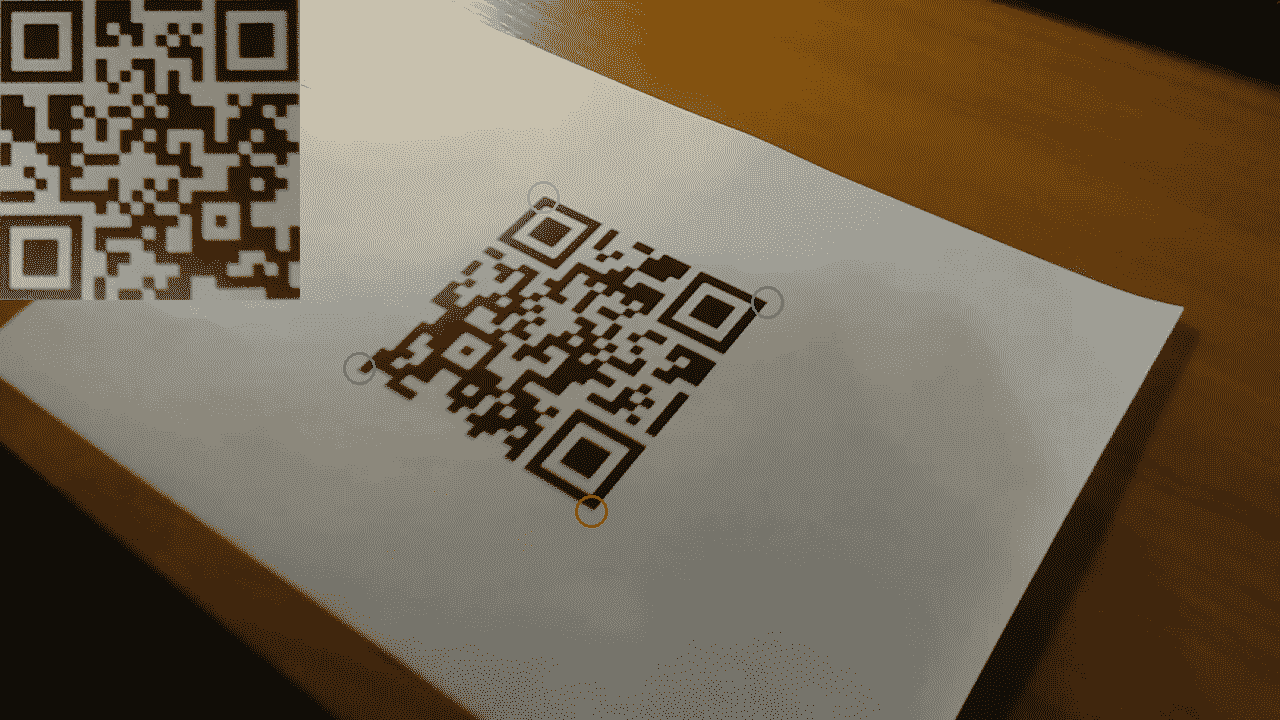# 四、目标检测与机器学习

• 使用 GrabCut 算法获取对象遮罩
• 使用 Canny 算法查找边缘
• 使用霍夫变换检测直线和圆
• 通过模板匹配查找对象
• 实时中值流对象跟踪器
• 通过跟踪 API 使用不同的算法跟踪对象
• 计算两个帧之间的密集光流
• 检测棋盘和圆形网格图案
• 使用 SVM 模型的简单行人探测器
• 使用不同的机器学习模型进行光学字符识别
• 使用 Haar/LBP 级联检测人脸
• 为 AR 应用检测 AruCo 模式
• 在自然场景中检测文字
• QR 码检测器和识别器

# 操作步骤

1. 导入模块：
import cv2
import numpy as np

1. 打开图像并定义鼠标回调函数以在图像上绘制一个矩形：
img = cv2.imread('../data/Lena.png', cv2.IMREAD_COLOR)
show_img = np.copy(img)

mouse_pressed = False
y = x = w = h = 0

def mouse_callback(event, _x, _y, flags, param):
global show_img, x, y, w, h, mouse_pressed

if event == cv2.EVENT_LBUTTONDOWN:
mouse_pressed = True
x, y = _x, _y
show_img = np.copy(img)

elif event == cv2.EVENT_MOUSEMOVE:
if mouse_pressed:
show_img = np.copy(img)
cv2.rectangle(show_img, (x, y),
(_x, _y), (0, 255, 0), 3)

elif event == cv2.EVENT_LBUTTONUP:
mouse_pressed = False
w, h = _x - x, _y - y

1. 显示图像，并在完成矩形并按下键盘上的A按钮之后，使用以下代码关闭窗口：
cv2.namedWindow('image')
cv2.setMouseCallback('image', mouse_callback)

while True:
cv2.imshow('image', show_img)
k = cv2.waitKey(1)

if k == ord('a') and not mouse_pressed:
if w*h > 0:
break

cv2.destroyAllWindows()

1. 调用cv2.grabCut基于绘制的矩形创建对象遮罩。 然后，创建对象掩码并将其定义为：
labels = np.zeros(img.shape[:2],np.uint8)

labels, bgdModel, fgdModel = cv2.grabCut(img, labels, (x, y, w, h), None, None, 5, cv2.GC_INIT_WITH_RECT)

show_img = np.copy(img)
show_img[(labels == cv2.GC_PR_BGD)|(labels == cv2.GC_BGD)] //= 3

cv2.imshow('image', show_img)
cv2.waitKey()
cv2.destroyAllWindows()

1. 定义鼠标回调以在图像上绘制遮罩。 有必要修复先前的cv2.grabCut调用中的错误：
label = cv2.GC_BGD
lbl_clrs = {cv2.GC_BGD: (0,0,0), cv2.GC_FGD: (255,255,255)}

def mouse_callback(event, x, y, flags, param):
global mouse_pressed

if event == cv2.EVENT_LBUTTONDOWN:
mouse_pressed = True
cv2.circle(labels, (x, y), 5, label, cv2.FILLED)
cv2.circle(show_img, (x, y), 5, lbl_clrs[label], cv2.FILLED)

elif event == cv2.EVENT_MOUSEMOVE:
if mouse_pressed:
cv2.circle(labels, (x, y), 5, label, cv2.FILLED)
cv2.circle(show_img, (x, y), 5, lbl_clrs[label], cv2.FILLED)

elif event == cv2.EVENT_LBUTTONUP:
mouse_pressed = False

1. 带遮罩显示图像； 使用白色绘制将对象像素标记为背景的位置，使用黑色绘制将背景区域标记为对象的位置。 然后，再次调用cv2.grabCut以获取固定的掩码。 最后，更新图像上的遮罩，并显示它：
cv2.namedWindow('image')
cv2.setMouseCallback('image', mouse_callback)

while True:
cv2.imshow('image', show_img)
k = cv2.waitKey(1)

if k == ord('a') and not mouse_pressed:
break
elif k == ord('l'):
label = cv2.GC_FGD - label

cv2.destroyAllWindows()

labels, bgdModel, fgdModel = cv2.grabCut(img, labels, None, bgdModel, fgdModel, 5, cv2.GC_INIT_WITH_MASK)

show_img = np.copy(img)
show_img[(labels == cv2.GC_PR_BGD)|(labels == cv2.GC_BGD)] //= 3

cv2.imshow('image', show_img)
cv2.waitKey()
cv2.destroyAllWindows()


# 工作原理

OpenCV 的cv2.grabCut实现了 GrabCut 算法。 此函数可以在多种模式下工作，并采用以下参数：输入 3 通道图像，带有像素初始标签的矩阵，（xywh）格式来定义标签初始化，两个用于存储进程状态的矩阵，多次迭代以及我们希望函数启动的模式。# 操作步骤

1. 导入模块：
import cv2
import matplotlib.pyplot as plt

1. 加载测试图像：
image = cv2.imread('../data/Lena.png')

1. 使用 Canny 算法检测边缘：
edges = cv2.Canny(image, 200, 100)

1. 可视化结果：
plt.figure(figsize=(8,5))
plt.subplot(121)
plt.axis('off')
plt.title('original')
plt.imshow(image[:,:,[2,1,0]])
plt.subplot(122)
plt.axis('off')
plt.title('edges')
plt.imshow(edges, cmap='gray')
plt.tight_layout()
plt.show()


# 工作原理

Canny 边缘检测是计算机视觉中非常强大且流行的工具。 它以 John F. Canny 的名字命名，他于 1986 年提出了该算法。OpenCV 在函数cv2.Canny中实现了该算法。 您必须在此函数中为梯度幅度指定两个阈值：第一个阈值用于检测强边缘，第二个阈值用于滞后过程，在该过程中将生长强边缘。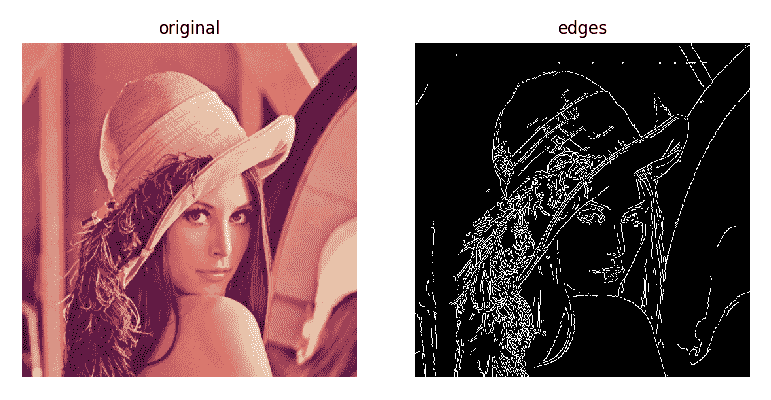# 操作步骤

1. 导入模块：
import cv2
import numpy as np

1. 绘制测试图像：
img = np.zeros((500, 500), np.uint8)
cv2.circle(img, (200, 200), 50, 255, 3)
cv2.line(img, (100, 400), (400, 350), 255, 3)

1. 使用概率霍夫变换检测线：
lines = cv2.HoughLinesP(img, 1, np.pi/180, 100, 100, 10)

1. 使用霍夫变换检测圆：
circles = cv2.HoughCircles(img, cv2.HOUGH_GRADIENT, 1, 15, param1=200, param2=30)

1. 绘制检测到的直线和圆：
dbg_img = np.zeros((img.shape, img.shape, 3), np.uint8)
for x1, y1, x2, y2 in lines:
print('Detected line: ({} {}) ({} {})'.format(x1, y1, x2, y2))
cv2.line(dbg_img, (x1, y1), (x2, y2), (0, 255, 0), 2)

for c in circles:
print('Detected circle: center=({} {}), radius={}'.format(c, c, c))
cv2.circle(dbg_img, (c, c), c, (0, 255, 0), 2)

1. 可视化结果：
plt.figure(figsize=(8,4))
plt.subplot(121)
plt.title('original')
plt.axis('off')
plt.imshow(img, cmap='gray')
plt.subplot(122)
plt.title('detected primitives')
plt.axis('off')
plt.imshow(dbg_img)
plt.show()


# 工作原理

Detected line: (99 401) (372 353)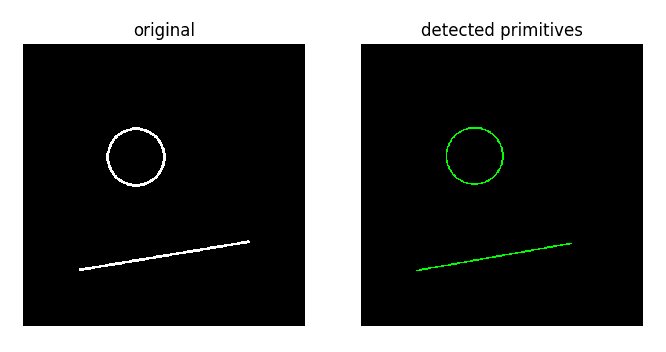# 操作步骤

1. 导入模块：
import cv2
import numpy as np

1. 加载图像并定义鼠标回调函数以选择图像 ROI。 所绘制矩形的内部将是我们用于匹配的模板：
img = cv2.imread('../data/Lena.png', cv2.IMREAD_COLOR)
show_img = np.copy(img)

mouse_pressed = False
y = x = w = h = 0

def mouse_callback(event, _x, _y, flags, param):
global show_img, x, y, w, h, mouse_pressed

if event == cv2.EVENT_LBUTTONDOWN:
mouse_pressed = True
x, y = _x, _y
show_img = np.copy(img)

elif event == cv2.EVENT_MOUSEMOVE:
if mouse_pressed:
show_img = np.copy(img)
cv2.rectangle(show_img, (x, y),
(_x, _y), (0, 255, 0), 2)

elif event == cv2.EVENT_LBUTTONUP:
mouse_pressed = False
w, h = _x - x, _y - y

1. 显示图像，用鼠标选择要查找的对象，然后按A按钮完成该过程并获取模板：
cv2.namedWindow('image')
cv2.setMouseCallback('image', mouse_callback)

while True:
cv2.imshow('image', show_img)
k = cv2.waitKey(1)

if k == ord('a') and not mouse_pressed:
if w*h > 0:
break

cv2.destroyAllWindows()

template = np.copy(img[y:y+h, x:x+w])

1. 显示图像并处理按钮按下事件。 从 0 到 5 的数字决定了我们用来在图像上查找与模板相似的区域的方法。 匹配通过cv2.matchTemplate函数执行。 匹配完成后，我们将找到具有最高（或最低）相似性度量的点，并得出检测结果：
methods = ['cv2.TM_CCOEFF', 'cv2.TM_CCOEFF_NORMED', 'cv2.TM_CCORR',
'cv2.TM_CCORR_NORMED', 'cv2.TM_SQDIFF', 'cv2.TM_SQDIFF_NORMED']

show_img = np.copy(img)

while True:
cv2.imshow('image', show_img)
k = cv2.waitKey()

if k == 27:
break
elif k > 0 and chr(k).isdigit():
index = int(chr(k))
if 0 <= index < len(methods):
method = methods[index]

res = cv2.matchTemplate(img, template, eval(method))

res = cv2.normalize(res, None, 0, 1, cv2.NORM_MINMAX)

if index >= methods.index('cv2.TM_SQDIFF'):
loc = np.where(res < 0.01)
else:
loc = np.where(res > 0.99)

show_img = np.copy(img)
for pt in zip(*loc[::-1]):
cv2.rectangle(show_img, pt, (pt + w, pt + h),
(0, 0, 255), 2)

res = cv2.resize(res, show_img.shape[:2])*255
res = cv2.cvtColor(res, cv2.COLOR_GRAY2BGR).astype(np.uint8)
cv2.putText(res, method, (0, 30), cv2.FONT_HERSHEY_SIMPLEX,
1, (0, 0, 255), 3)

show_img = np.hstack((show_img, res))

cv2.destroyAllWindows()


# 工作原理

cv2.matchTemplate用于查找与模板相似的图像区域。 可以使用不同的方法（通过不同的数学运算来确定模板和图像上的色块之间的差异）来确定相似性。 但是，这些方法都无法找到具有不同比例或方向的模板。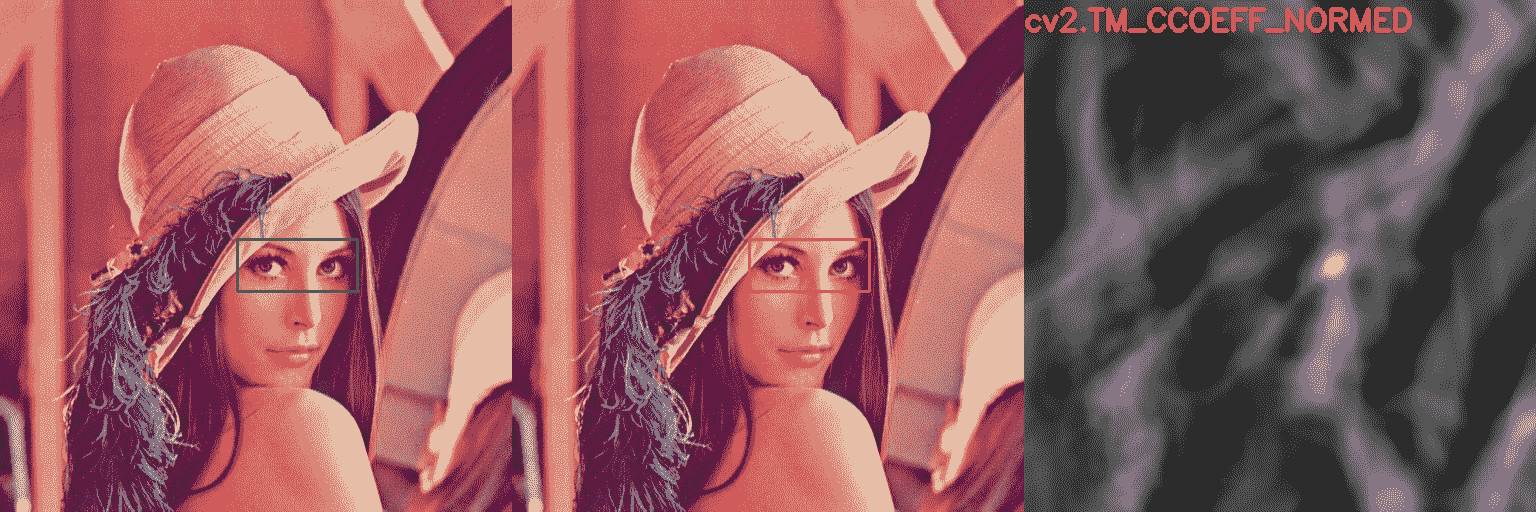# 操作步骤

1. 导入所有必要的模块：
import cv2
import numpy as np

1. 打开视频文件，读取其框架，然后选择要跟踪的对象：
cap = cv2.VideoCapture("../data/traffic.mp4")

bbox = cv2.selectROI(frame, False, True)

cv2.destroyAllWindows()

1. 创建 Median Flow 跟踪器，并使用视频中的第一帧和我们选择的边界框对其进行初始化。 然后，一一读取剩余的帧，将它们输入到跟踪器中，并为每个帧获得一个新的边框。 显示边界框，以及“中值流”算法每秒能够处理的帧数：
tracker = cv2.TrackerMedianFlow_create()
status_tracker = tracker.init(frame, bbox)
fps = 0

while True:
if not status_cap:
break

if status_tracker:
timer = cv2.getTickCount()
status_tracker, bbox = tracker.update(frame)

if status_tracker:
x, y, w, h = [int(i) for i in bbox]
cv2.rectangle(frame, (x, y), (x + w, y + h), (0, 255, 0), 15)
fps = cv2.getTickFrequency() / (cv2.getTickCount() - timer);
cv2.putText(frame, "FPS: %.0f" % fps, (0, 80), cv2.FONT_HERSHEY_SIMPLEX, 3.5, (0, 0, 0), 8);
else:
cv2.putText(frame, "Tracking failure detected", (0, 80), cv2.FONT_HERSHEY_SIMPLEX, 3.5, (0,0,255), 8)

cv2.imshow("MedianFlow tracker", frame)

k = cv2.waitKey(1)

if k == 27:
break

cv2.destroyAllWindows()


# 工作原理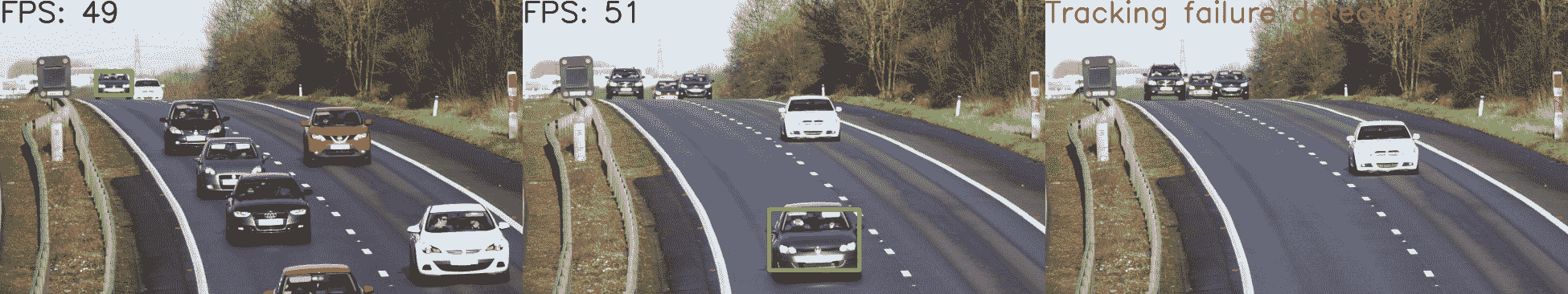# 操作步骤

1. 导入模块：
import cv2

1. 创建主窗口并在不同的跟踪器上循环：
cv2.namedWindow('frame')

for name, tracker in (('KCF', cv2.TrackerKCF_create),
('MIL', cv2.TrackerMIL_create),
('TLD', cv2.TrackerTLD_create)):
tracker = tracker()
initialized = False

1. 打开测试视频文件，然后选择一个对象：
video = cv2.VideoCapture('../data/traffic.mp4')
bbox = (878, 266, 1153-878, 475-266)

1. 跟踪直到视频结束或按下Esc，并可视化当前跟踪的对象：
    while True:
t0 = time.time()
if not ok:
break

if initialized:
tracked, bbox = tracker.update(frame)
else:
cv2.imwrite('/tmp/frame.png', frame)
tracked = tracker.init(frame, bbox)
initialized = True

fps = 1 / (time.time() - t0)
cv2.putText(frame, 'tracker: {}, fps: {:.1f}'.format(name, fps),
(10, 50), cv2.FONT_HERSHEY_SIMPLEX, 1, (255, 0, 0), 2)
if tracked:
bbox = tuple(map(int, bbox))
cv2.rectangle(frame, (bbox, bbox),
(bbox+bbox, bbox+bbox),
(0, 255, 0), 3)
cv2.imshow('frame', frame)
if cv2.waitKey(3) == 27:
break

1. 关闭窗口：
cv2.destroyAllWindows()


# 工作原理

OpenCV 跟踪 API 提供对许多不同跟踪算法的访问，例如中位数流，核化相关过滤器KCF），跟踪学习检测TLD）等。 可以通过cv2.TrackerKCF_create方法实例化跟踪器（可以代替 KCF 来指定任何其他受支持的跟踪算法名称）。 必须为第一帧初始化跟踪模型，并使用方法tracker.init指定初始对象位置。 之后，必须使用tracker.update方法处理每个帧，该方法将返回跟踪状态和被跟踪对象的当前位置。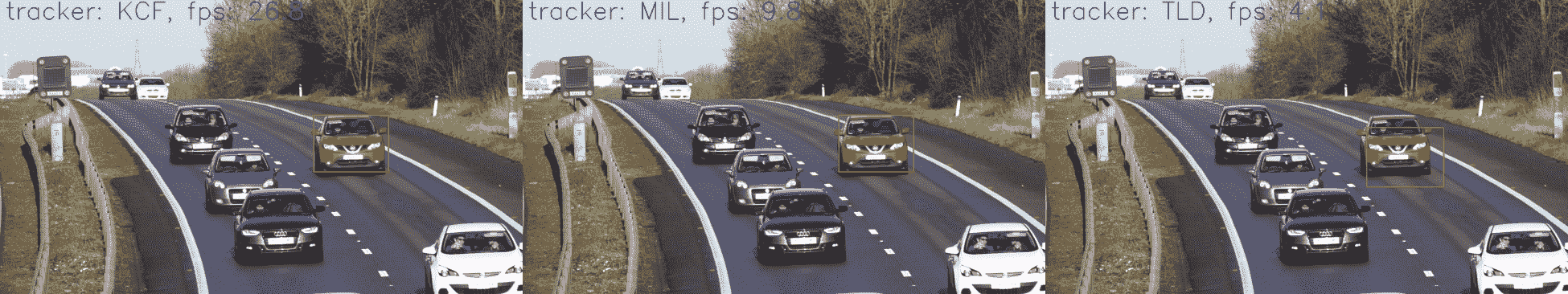# 操作步骤

1. 导入我们将要使用的模块：
import cv2
import numpy as np

1. 定义函数以显示光流：
def display_flow(img, flow, stride=40):
for index in np.ndindex(flow[::stride, ::stride].shape[:2]):
pt1 = tuple(i*stride for i in index)
delta = flow[pt1].astype(np.int32)[::-1]
pt2 = tuple(pt1 + 10*delta)
if 2 <= cv2.norm(delta) <= 10:
cv2.arrowedLine(img, pt1[::-1], pt2[::-1], (0,0,255), 5, cv2.LINE_AA, 0, 0.4)

norm_opt_flow = np.linalg.norm(flow, axis=2)
norm_opt_flow = cv2.normalize(norm_opt_flow, None, 0, 1, cv2.NORM_MINMAX)

cv2.imshow('optical flow', img)
cv2.imshow('optical flow magnitude', norm_opt_flow)
k = cv2.waitKey(1)

if k == 27:
return 1
else:
return 0

1. 打开视频并获取其第一帧。 接下来，逐帧读取帧，并使用 Gunnar Farneback 的算法计算密集的光流。 然后，显示结果：
cap = cv2.VideoCapture("../data/traffic.mp4")

prev_frame = cv2.cvtColor(prev_frame, cv2.COLOR_BGR2GRAY)
prev_frame = cv2.resize(prev_frame, (0,0), None, 0.5, 0.5)
init_flow = True

while True:
frame = cv2.resize(frame, (0,0), None, 0.5, 0.5)
if not status_cap:
break
gray = cv2.cvtColor(frame, cv2.COLOR_BGR2GRAY)

if init_flow:
opt_flow = cv2.calcOpticalFlowFarneback(prev_frame, gray, None,
0.5, 5, 13, 10, 5, 1.1,
cv2.OPTFLOW_FARNEBACK_GAUSSIAN)
init_flow = False
else:
opt_flow = cv2.calcOpticalFlowFarneback(prev_frame, gray, opt_flow,
0.5, 5, 13, 10, 5, 1.1,
cv2.OPTFLOW_USE_INITIAL_FLOW)

prev_frame = np.copy(gray)

if display_flow(frame, opt_flow):
break;

cv2.destroyAllWindows()

1. 将视频捕获的位置设置为开始，然后读取第一帧。 创建一个可计算 Dual TV L1 光流的类的实例。 然后，一帧一帧地读取帧，并获取随后每对帧的光通量； 显示结果：
cap.set(cv2.CAP_PROP_POS_FRAMES, 0)

prev_frame = cv2.cvtColor(prev_frame, cv2.COLOR_BGR2GRAY)
prev_frame = cv2.resize(prev_frame, (0,0), None, 0.5, 0.5)

flow_DualTVL1 = cv2.createOptFlow_DualTVL1()

while True:
frame = cv2.resize(frame, (0,0), None, 0.5, 0.5)
if not status_cap:
break
gray = cv2.cvtColor(frame, cv2.COLOR_BGR2GRAY)

if not flow_DualTVL1.getUseInitialFlow():
opt_flow = flow_DualTVL1.calc(prev_frame, gray, None)
flow_DualTVL1.setUseInitialFlow(True)
else:
opt_flow = flow_DualTVL1.calc(prev_frame, gray, opt_flow)

prev_frame = np.copy(gray)

if display_flow(frame, opt_flow):
break;

cv2.destroyAllWindows()


# 工作原理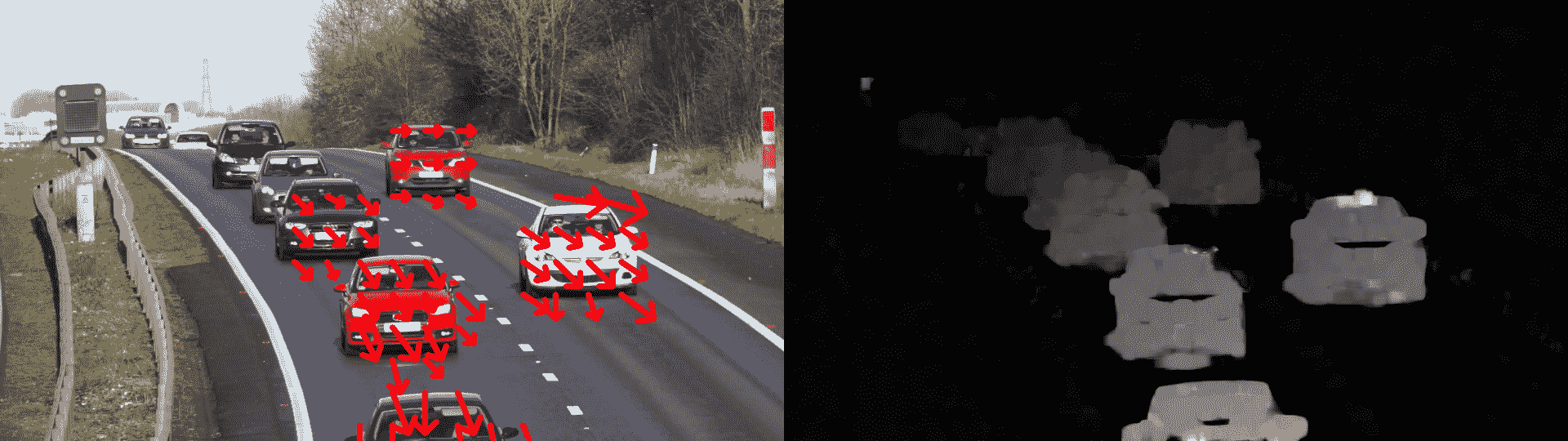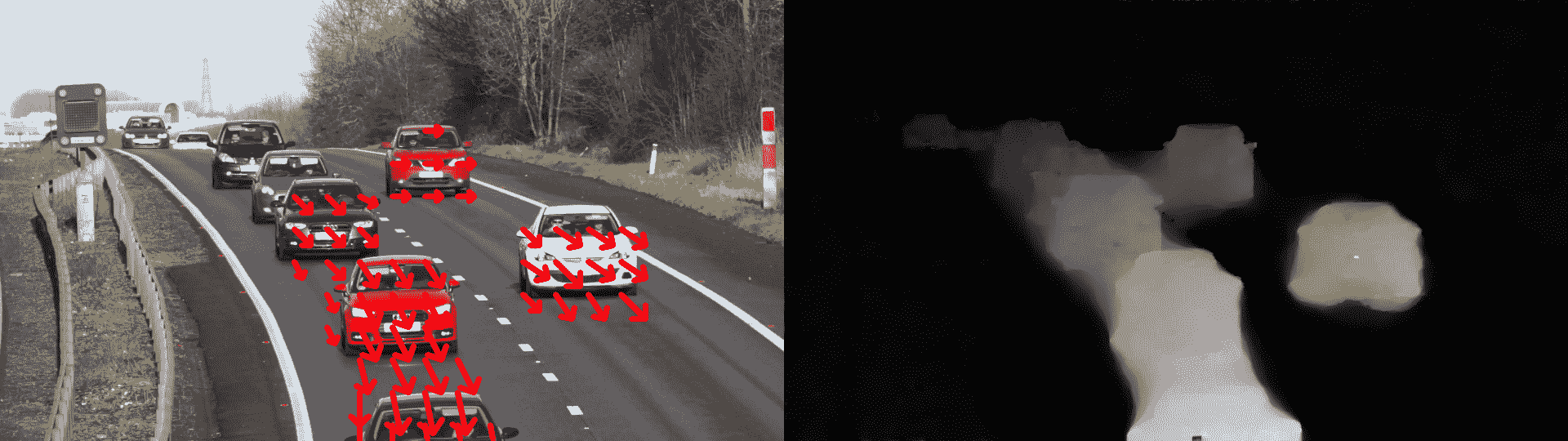# 操作步骤

1. 导入模块：
import cv2
import matplotlib.pyplot as plt

1. 用棋盘加载测试图像：
image_chess = cv2.imread('../data/chessboard.png')

1. 检测棋盘图案：
found, corners = cv2.findChessboardCorners(image_chess, (6, 9))
assert found == True, "can't find chessboard pattern"

1. 绘制检测到的图案：
dbg_image_chess = image_chess.copy()
cv2.drawChessboardCorners(dbg_image_chess, (6, 9), corners, found);

1. 使用圆形网格图案加载测试图像：
image_circles = cv2.imread('../data/circlesgrid.png')

1. 检测圆形网格图案：
found, corners = cv2.findCirclesGrid(image_circles, (6, 6), cv2.CALIB_CB_SYMMETRIC_GRID)
assert found == True, "can't find circles grid pattern"

1. 绘制检测到的图案：
dbg_image_circles = image_circles.copy()
cv2.drawChessboardCorners(dbg_image_circles, (6, 6), corners, found);

1. 可视化结果：
plt.figure(figsize=(8,8))
plt.subplot(221)
plt.title('original')
plt.axis('off')
plt.imshow(image_chess)
plt.subplot(222)
plt.title('detected pattern')
plt.axis('off')
plt.imshow(dbg_image_chess)
plt.show()
plt.subplot(223)
plt.title('original')
plt.axis('off')
plt.imshow(image_circles)
plt.subplot(224)
plt.title('detected pattern')
plt.axis('off')
plt.imshow(dbg_image_circles)
plt.tight_layout()
plt.show()


# 工作原理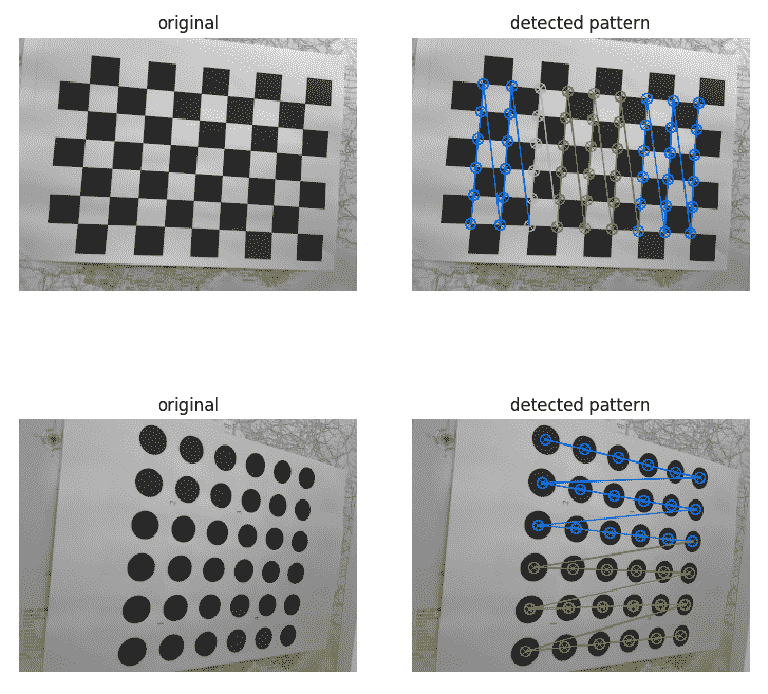# 操作步骤

1. 导入模块：
import cv2
import matplotlib.pyplot as plt

1. 加载测试图像：
image = cv2.imread('../data/people.jpg')

1. 创建 HOG 特征计算机和检测器：
hog = cv2.HOGDescriptor()
hog.setSVMDetector(cv2.HOGDescriptor_getDefaultPeopleDetector())

1. 检测图像中的人：
locations, weights = hog.detectMultiScale(image)

1. 绘制检测到的人物边界框：
dbg_image = image.copy()
for loc in locations:
cv2.rectangle(dbg_image, (loc, loc),
(loc+loc, loc+loc), (0, 255, 0), 2)

1. 可视化结果：
plt.figure(figsize=(12,6))
plt.subplot(121)
plt.title('original')
plt.axis('off')
plt.imshow(image[:,:,[2,1,0]])
plt.subplot(122)
plt.title('detections')
plt.axis('off')
plt.imshow(dbg_image[:,:,[2,1,0]])
plt.tight_layout()
plt.show()


# 工作原理

OpenCV 在类cv2.HOGDescriptor中实现定向直方图HOG）描述符计算功能。 可以使用线性 SVM 模型将同一类用于对象检测。 实际上，它已经具有带有权重的预训练行人检测器模型。 可以通过cv2.HOGDescriptor.getDefaultPeopleDetector方法获得模型。 使用hog.detectMultiScale方法，使用滑动窗口方法以多个比例检测对象。 该函数返回检测到的人的位置列表以及每个检测分数。 要了解更多信息，请访问这里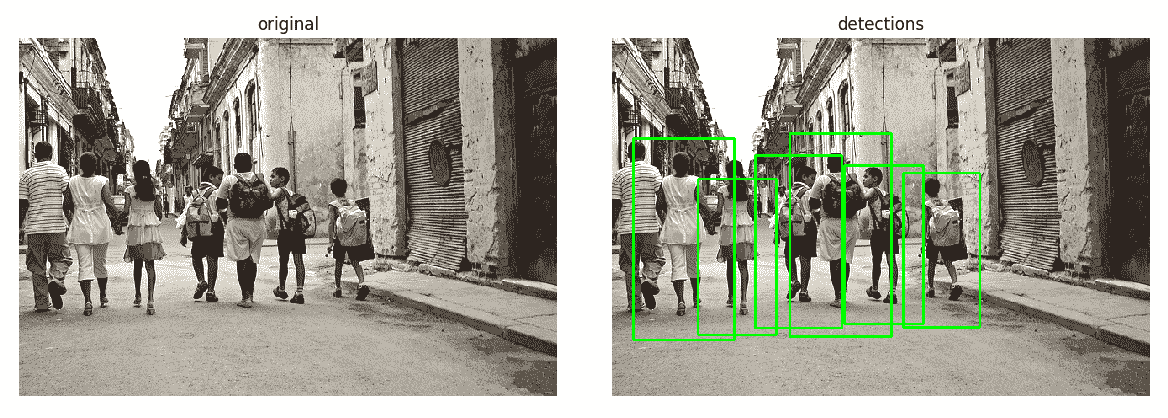# 操作步骤

1. 导入模块：
import cv2
import numpy as np

1. 指定一些常量：
CELL_SIZE = 20     # Digit image size.
NCLASSES = 10      # Number of digits.
TRAIN_RATIO = 0.8  # Part of all samples used for training.

1. 读取数字图像并准备标签：
digits_img = cv2.imread('../data/digits.png', 0)
digits = [np.hsplit(r, digits_img.shape // CELL_SIZE)
for r in np.vsplit(digits_img, digits_img.shape // CELL_SIZE)]
digits = np.array(digits).reshape(-1, CELL_SIZE, CELL_SIZE)
nsamples = digits.shape
labels = np.repeat(np.arange(NCLASSES), nsamples // NCLASSES)

1. 执行几何归一化，计算图像矩并对齐每个样本：
for i in range(nsamples):
m = cv2.moments(digits[i])
if m['mu02'] > 1e-3:
s = m['mu11'] / m['mu02']
M = np.float32([[1, -s, 0.5*CELL_SIZE*s],
[0, 1, 0]])
digits[i] = cv2.warpAffine(digits[i], M, (CELL_SIZE, CELL_SIZE))

1. 随机排列样本：
perm = np.random.permutation(nsamples)
digits = digits[perm]
labels = labels[perm]

1. 定义用于计算 HOG 描述符的函数：
def calc_hog(digits):
win_size = (20, 20)
block_size = (10, 10)
block_stride = (10, 10)
cell_size = (10, 10)
nbins = 9
hog = cv2.HOGDescriptor(win_size, block_size, block_stride, cell_size, nbins)
samples = []
for d in digits: samples.append(hog.compute(d))
return np.array(samples, np.float32)

1. 准备训练和测试数据（特征和标签）：
ntrain = int(TRAIN_RATIO * nsamples)
fea_hog_train = calc_hog(digits[:ntrain])
fea_hog_test = calc_hog(digits[ntrain:])
labels_train, labels_test = labels[:ntrain], labels[ntrain:]

1. 创建一个 KNN 模型：
K = 3
knn_model = cv2.ml.KNearest_create()
knn_model.train(fea_hog_train, cv2.ml.ROW_SAMPLE, labels_train)

1. 创建一个 SVM 模型：
svm_model = cv2.ml.SVM_create()
svm_model.setGamma(2)
svm_model.setC(1)
svm_model.setKernel(cv2.ml.SVM_RBF)
svm_model.setType(cv2.ml.SVM_C_SVC)
svm_model.train(fea_hog_train, cv2.ml.ROW_SAMPLE, labels_train)

1. 定义评估模型的函数：
def eval_model(fea, labels, fpred):
pred = fpred(fea).astype(np.int32)
acc = (pred.T == labels).mean()*100

conf_mat = np.zeros((NCLASSES, NCLASSES), np.int32)
for c_gt, c_pred in zip(labels, pred):
conf_mat[c_gt, c_pred] += 1

return acc, conf_mat

1. 评估 KNN 和 SVM 模型：
knn_acc, knn_conf_mat = eval_model(fea_hog_test, labels_test, lambda fea: knn_model.findNearest(fea, K))
print('KNN accuracy (%):', knn_acc)
print('KNN confusion matrix:')
print(knn_conf_mat)

svm_acc, svm_conf_mat = eval_model(fea_hog_test, labels_test, lambda fea: svm_model.predict(fea))
print('SVM accuracy (%):', svm_acc)
print('SVM confusion matrix:')
print(svm_conf_mat)


# 工作原理

KNN accuracy (%): 91.1
KNN confusion matrix:
[[101   0   0   0   0   0   1   0   0   2]
[  0 112   3   0   0   0   0   0   0   0]
[  0   1  93   1   0   0   0   0   2   0]
[  1   0   3 100   0   3   0   0   1   1]
[  1   0   2   8  78   3   4   0   1   5]
[  0   0   0   5   0  82   1   0   4   1]
[  0   0   0   0   1   0  92   0   0   0]
[  0   0   3   6   2   1   0  76   1   2]
[  0   0   0   1   0   2   0   1  80   2]
[  2   1   1   1   0   0   0   4   4  97]]

SVM accuracy (%): 93.5
SVM confusion matrix:
[[100   0   1   0   0   0   1   0   0   2]
[  0 112   2   0   0   0   0   1   0   0]
[  0   0  93   0   1   0   0   1   2   0]
[  1   0   2 100   0   2   0   1   2   1]
[  1   0   1   2  93   2   0   1   0   2]
[  0   0   0   3   1  85   1   1   2   0]
[  0   0   0   0   1   0  92   0   0   0]
[  0   0   1   3   3   2   0  82   0   0]
[  2   0   0   1   0   2   0   0  79   2]
[  1   1   1   1   1   1   0   4   1  99]]


# 操作步骤

1. 导入我们需要的模块：
import cv2
import numpy as np

1. 定义打开视频文件，调用检测器以查找图像中所有面部并显示结果的函数：
def detect_faces(video_file, detector, win_title):
cap = cv2.VideoCapture(video_file)

while True:
if not status_cap:
break

gray = cv2.cvtColor(frame, cv2.COLOR_BGR2GRAY)

faces = detector.detectMultiScale(gray, 1.3, 5)

for x, y, w, h in faces:
cv2.rectangle(frame, (x, y), (x + w, y + h), (0, 255, 0), 3)
text_size, _ = cv2.getTextSize('Face', cv2.FONT_HERSHEY_SIMPLEX, 1, 2)
cv2.rectangle(frame, (x, y - text_size), (x + text_size, y), (255, 255, 255), cv2.FILLED)
cv2.putText(frame, 'Face', (x, y), cv2.FONT_HERSHEY_SIMPLEX, 1, (0, 0, 0), 2)
cv2.imshow(win_title, frame)

if cv2.waitKey(1) == 27:
break

cv2.destroyAllWindows()

1. 从 OpenCV 加载经过预训练的 Haar 级联，然后调用我们的检测函数：
haar_face_cascade = cv2.CascadeClassifier('../data/haarcascade_frontalface_default.xml')


1. 以略有不同的方式加载预训练的 LBP 级联，然后再次调用该函数以查找和显示面部：
lbp_face_cascade = cv2.CascadeClassifier()



# 工作原理

OpenCV 包含许多用于不同目的的经过预先训练的检测器，您可以找到猫，眼睛，车牌，身体，当然还有人脸的边界框。 所有这些检测器都可以在 OpenCV 主存储库的/data子目录中找到。 所有检测器均以.xml文件表示，该文件包含检测器的所有参数。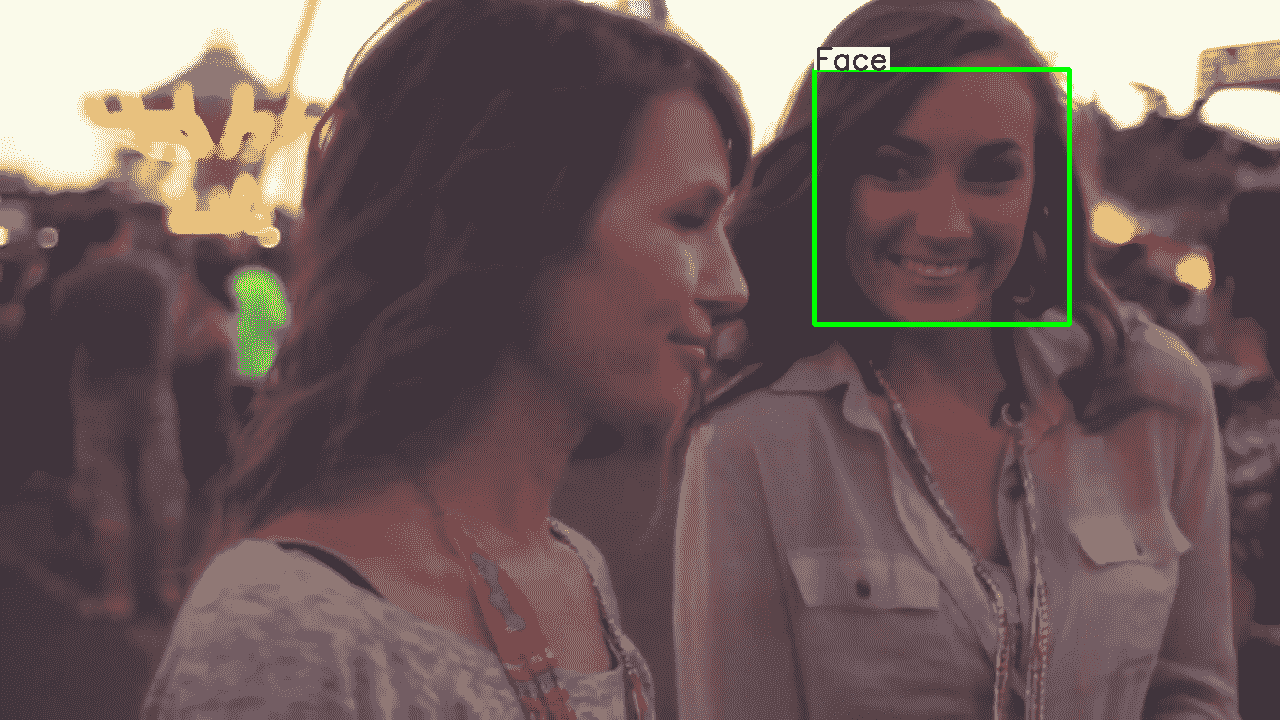# 操作步骤

1. 导入模块：
import cv2
import cv2.aruco as aruco
import numpy as np

1. 使用不同的 AruCo 标记创建图像，对其进行模糊处理，然后显示它：
aruco_dict = aruco.getPredefinedDictionary(aruco.DICT_6X6_250)

img = np.full((700, 700), 255, np.uint8)

img[100:300, 100:300] = aruco.drawMarker(aruco_dict, 2, 200)
img[100:300, 400:600] = aruco.drawMarker(aruco_dict, 76, 200)
img[400:600, 100:300] = aruco.drawMarker(aruco_dict, 42, 200)
img[400:600, 400:600] = aruco.drawMarker(aruco_dict, 123, 200)

img = cv2.GaussianBlur(img, (11, 11), 0)

cv2.imshow('Created AruCo markers', img)
cv2.waitKey(0)
cv2.destroyAllWindows()

1. 检测模糊图像上的标记。 绘制检测到的标记并显示结果：
aruco_dict = aruco.getPredefinedDictionary(aruco.DICT_6X6_250)

corners, ids, _ = aruco.detectMarkers(img, aruco_dict)

img_color = cv2.cvtColor(img, cv2.COLOR_GRAY2BGR)
aruco.drawDetectedMarkers(img_color, corners, ids)

cv2.imshow('Detected AruCo markers', img_color)
cv2.waitKey(0)
cv2.destroyAllWindows()


# 工作原理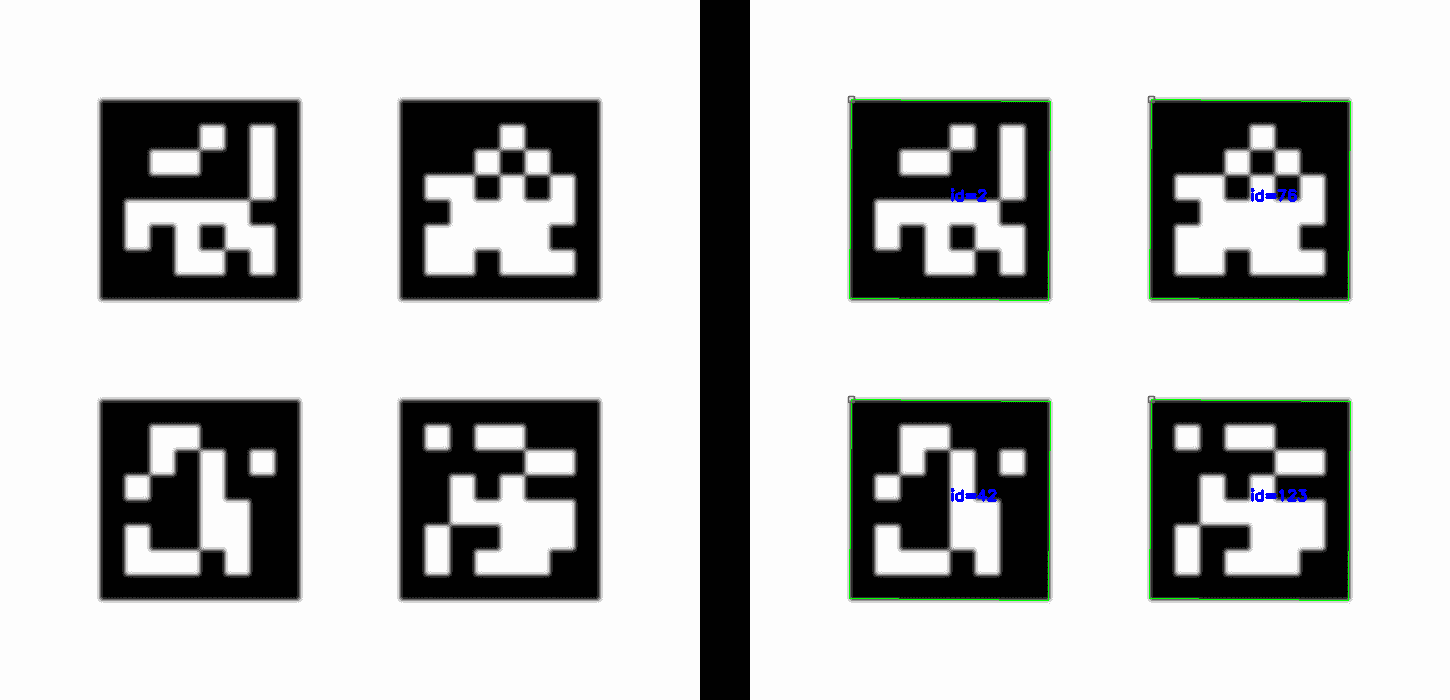# 操作步骤

1. 导入模块：
import cv2

1. 加载文字图片：
img = cv2.imread('../data/scenetext01.jpg')

1. 加载预训练的卷积神经网络并检测文本消息：
det = cv2.text.TextDetectorCNN_create(
"../data/textbox.prototxt", "../data/TextBoxes_icdar13.caffemodel")
rects, probs = det.detect(img)

1. 绘制置信度高于阈值的检测到的文本边界框：
THR = 0.3
for i, r in enumerate(rects):
if probs[i] > THR:
cv2.rectangle(img, (r, r), (r+r, r+r), (0, 255, 0), 2)

1. 可视化结果：
plt.figure(figsize=(10,8))
plt.axis('off')
plt.imshow(img[:,:,[2,1,0]])
plt.tight_layout()
plt.show()


# 工作原理

OpenCV 中实现了许多不同的文本检测方法。 在本秘籍中，您学习了如何使用最新的深度学习方法来检测文本边界框。 OpenCV 类cv2.TextDetectorCNN_create创建一个 CNN（卷积神经网络）模型，并从指定的文件加载其预先训练的权重。 在那之后，您只需要调用det.detect方法，该方法将返回一个矩形列表以及包含文本的矩形的相关概率。# 操作步骤

1. 导入我们需要的模块：
import cv2
import numpy as np

1. 实现一个找到两条线的交点的函数：
def intersect(l1, l2):
delta = np.array([l1 - l1, l2 - l2]).astype(np.float32)

delta = 1 / delta
delta[:, 0] *= -1

b = np.matmul(delta, np.array([l1, l2]).transpose())
b = np.diagonal(b).astype(np.float32)

res = cv2.solve(delta, b)
return res, tuple(res.astype(np.int32).reshape((2)))

1. 定义一个函数，该函数通过计算四对变形点和非变形点之间的对应关系来消除透视变形：
def rectify(image, corners, out_size):
rect = np.zeros((4, 2), dtype = "float32")
rect = corners
rect = corners
rect = corners
rect = corners

dst = np.array([
[0, 0],
[out_size - 1, 0],
[out_size - 1, out_size - 1],
[0, out_size - 1]], dtype = "float32")

M = cv2.getPerspectiveTransform(rect, dst)
rectified = cv2.warpPerspective(image, M, out_size)
return rectified

1. 创建一个查找 QR 码外角的函数：
def qr_code_outer_corners(image):
outer_corners_found = False
outer_corners = []

gray = cv2.cvtColor(image, cv2.COLOR_BGR2GRAY)
_, th = cv2.threshold(gray, 0, 255, cv2.THRESH_BINARY + cv2.THRESH_OTSU)

_, contours, hierarchy = \
cv2.findContours(th, cv2.RETR_TREE, cv2.CHAIN_APPROX_SIMPLE)

cnts = []
centers = []

hierarchy = hierarchy.reshape((-1, 4))
for i in range(hierarchy.shape):
i_next, i_prev, i_child, i_par = hierarchy[i]
if all(v == -1 for v in hierarchy[i][:3]):
if all(v == -1 for v in hierarchy[i_par][:2]):
ids = [i, i_par, hierarchy[i_par]]
corner_cnts = []
for id_ in ids:
cnt = contours[id_]
apprx = \
cv2.approxPolyDP(cnt, cv2.arcLength(cnt, True) * 0.02, True)
if len(apprx) == 4:
corner_cnts.append(apprx.reshape((4, -1)))
if len(corner_cnts) == 3:
cnts.append(corner_cnts)
all_pts = np.array(corner_cnts).reshape(-1, 2)

centers.append(np.mean(all_pts, 0))

if len(centers) == 3:
distances_between_pts = np.linalg.norm(np.roll(centers, 1, 0) - centers, axis=1)
max_dist_id = np.argmax(distances_between_pts)

index_diag_pt_1 = max_dist_id
index_diag_pt_2 = (max_dist_id - 1) % len(centers)
index_corner_pt = (len(centers) - 1)*len(centers) // 2 - index_diag_pt_1 - index_diag_pt_2

middle_pt = 0.5 * (centers[index_diag_pt_1] + centers[index_diag_pt_2])

i_ul_pt = np.argmax(np.linalg.norm(cnts[index_corner_pt][-1] - middle_pt, axis=1))
ul_pt = cnts[index_corner_pt][-1][i_ul_pt]

for i in [index_diag_pt_1, index_diag_pt_2]:
corner_cnts = cnts[i]
outer_cnt = corner_cnts[-1]

distances_to_mp = np.linalg.norm(outer_cnt - middle_pt, axis=1)
max_dist_id = np.argmax(distances_to_mp)

vec_from_mid_to_diag = outer_cnt[max_dist_id] - middle_pt
vec_from_mid_to_corner = ul_pt - middle_pt
cross_prod = np.cross(vec_from_mid_to_corner, vec_from_mid_to_diag)

diff_idx = 0

if cross_prod > 0:
ur_pt = outer_cnt[max_dist_id]
ur_pt_2 = outer_cnt[(max_dist_id + 1) % len(outer_cnt)]
else:
bl_pt = outer_cnt[max_dist_id]
bl_pt_2 = outer_cnt[(max_dist_id - 1) % len(outer_cnt)]

ret, br_pt = intersect((bl_pt, bl_pt_2), (ur_pt, ur_pt_2))

if ret == True:
outer_corners_found = True
outer_corners = [ul_pt, ur_pt, br_pt, bl_pt]

return outer_corners_found, outer_corners

1. 打开带有 QR 码的视频，在每个帧上找到 QR 码，如果成功，则显示代码角并取消扭曲代码以获取规范视图：
cap = cv2.VideoCapture('../data/qr.mp4')

while True:
if ret == False:
break

result, corners = qr_code_outer_corners(frame)

qr_code_size = 300

if result:
if all((0, 0) < tuple(c) < (frame.shape, frame.shape) for c in corners):
rectified = rectify(frame, corners, (qr_code_size, qr_code_size))

cv2.circle(frame, tuple(corners), 15, (0, 255, 0), 2)
cv2.circle(frame, tuple(corners), 15, (0, 0, 255), 2)
cv2.circle(frame, tuple(corners), 15, (255, 0, 0), 2)
cv2.circle(frame, tuple(corners), 15, (255, 255, 0), 2)

frame[0:qr_code_size, 0:qr_code_size] = rectified

cv2.imshow('QR code detection', frame)

k = cv2.waitKey(100)

if k == 27:
break

cap.release()
cv2.destroyAllWindows()


# 工作原理